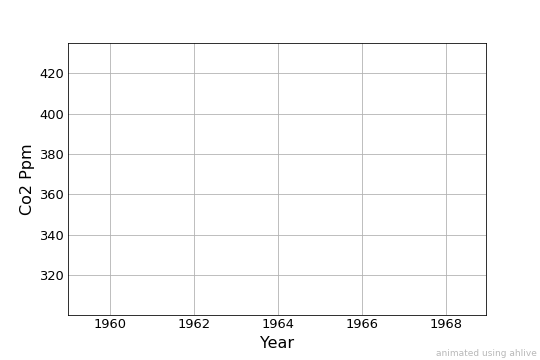# Overview¶

Get acquainted with ahlive!

## easy as 1-2-3-4¶

With just a few lines of code, create a stimulating animation!

:

import ahlive as ah
df = ah.open_dataset(label='annual_co2')
ah_df = ah.DataFrame(df, xs='year', ys='co2_ppm')
ah_df.render()

ANNUAL CO2 | Source: NOAA ESRL | https://www.esrl.noaa.gov/
[########################################] | 100% Completed | 16.9s

:## simply annotate¶

Conveniently add dynamic limits, labels, references, and remarks.

:

import ahlive as ah
df = ah.open_dataset(label="annual_co2")
ah_df = ah.DataFrame(
df,
xs="year",
ys="co2_ppm",
ylim1s='explore_0.05',
ylabel="CO2 [ppm]",
state_labels="year",
inline_labels="co2_ppm",
title="Annual Mean CO2 Concentration",
)
ah_df = ah_df.reference(x0s='x', label='')
ah_df = ah_df.remark(xs=2000, durations=1, remarks="Year 2000")
ah_df = ah_df.config('inline', suffix=' ppm')
ah_df.render()

ANNUAL CO2 | Source: NOAA ESRL | https://www.esrl.noaa.gov/
[########################################] | 100% Completed | 19.9s

: